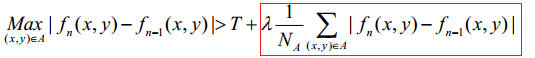# Python基於OpenCV的視頻圖像處理詳解

## 初識OpenCV

OpenCV是一個開源的，跨平臺的計算機視覺庫，它采用優化的C/C++代碼編寫，能夠充分利用多核處理器的優勢，提供瞭Python，Ruby，MATPLOAB以及其他高級語言接口。

OpenCV的設計目標是執行速度盡量快，主要面向實時應用，是視頻信號處理的主要工具之一，它封裝瞭豐富的視頻處理相關的工具包。視頻信號是重要的視覺信息來源，其中包含的信息要遠大於圖像，對視頻的分析也是計算機視覺領域的重要研究方向之一。視頻在本質上由連鎖的多幀圖像構成，因此，視頻信號處理最終仍歸屬圖像處理范疇。但在視頻中，其時間維度也包含瞭許多有用的信息。

## 視頻讀寫處理

```import cv2
cap=cv2.VideoCapture('D:\Image\Funny.mp4')
while(cap.isOpened()):
gray=cv2.cvtColor(frame,cv2.COLOR_BGR2GRAY) #將原幀圖像轉化為灰度圖像
cv2.imshow('視頻捕捉',gray) #顯示處理後的圖像
if cv2.waitKey(1) & 0xFF==ord('q'): #按q鍵退出程序
break
cap.release() #處理完成，釋放視頻捕捉
cv2.destroyAllWindows() #關閉窗口釋放資源```由於視頻過長，原視頻放在瞭主頁。

```#攝像頭獲取視頻
import cv2
cap=cv2.VideoCapture(1) #打開攝像頭獲取視頻
while(True):
gray=cv2.cvtColor(frame,cv2.COLOR_BGR2GRAY)
#顯示結果
cv2.imshow('攝像頭拍照',gray)
if cv2.waitKey(1) & 0xFF==ord('q'):
break
cap.release()#處理完成，釋放視頻捕捉
cv2.destroyAllWindows()```

• ①.VideoWriter_fourcc('T','4','2','0'),該選項為一個未壓縮的YUV顏色編碼類型，是4：2：0色度子采樣。改編碼有著很好的兼容性，但是會產生較大的文件，文件拓展名為：“.avi”
• ②.VideoWriter_fourcc('P','T','M','1'):該選項為“MPEG-1”編碼類型，文件拓展名為“.mpeg”
• ③.VideoWrite_fourcc('X','V','T','D')：該選項是“MPEG-4”編碼類型，如果希望得到的視頻大小為平均值，推薦使用該選項，文件拓展名為：“.mp4”
• ④.VideWriter_fourcc('T','H','E','O'):該選項是“Ogg Vorbis”編碼類型，文件拓展名為“.ogv”。
• ⑤.VideWriter_foucc('F','L','V','T'):該選項是Flash編碼類型，文件拓展名是“.flv”。

```#通過OpenCv獲取視頻並寫入
import cv2
cap2=cv2.VideoCapture('D:\Image\Funny.mp4')
cap=cv2.VideoCapture(1)#打開攝像頭並獲取視頻
#對視頻幀率fps進行賦值
fps=24
#過去視頻幀的大小
size=(int(cap2.get(cv2.CAP_PROP_FRAME_WIDTH)),int(cap2.get(cv2.CAP_PROP_FRAME_HEIGHT)))
fourcc=cv2.VideoWriter_fourcc('X','V','I','D')  #MP4文件格式
out=cv2.VideoWriter('D:\Image\output.avi',fourcc,fps,size)#定義視頻文件寫入對象
while(cap.isOpened()):
if ret==True:
'''
獲取幀圖像並翻轉，cv2.flip()的第二個參數表示翻轉方式：0代表垂直翻轉，1代表水平翻轉，-1代表水平垂直翻轉
'''
frame=cv2.flip(frame,1)
out.write(frame)#將翻轉後的幀圖像寫入文件
cv2.imshow('幀圖像處理',frame)
if cv2.waitKey(1) & 0xFF==ord('q'):
break
else:
break
cap.release()
out.release()
cv2.destroyAllWindows()```

## 運動軌跡標記

```#L-K算法
import numpy as np
import cv2
#設置L-K算法參數
lk_params=dict(winSize=(15,15), #搜索窗口的大小
maxLevel=2,#最大金字塔層數
#迭代算法終止條件（迭代次數或迭代閾值）
criteria=(cv2.TERM_CRITERIA_EPS|cv2.TERM_CRITERIA_COUNT,10,0.03))
feature_params=dict(maxCorners=500,   #設置最多返回的關鍵點（角點）數
qualityLevel=0.3,  #角點閾值：反映一個像素點對強才算一個角點
minDistance=7, #角點之間的最小像素點（歐氏距離）
blockSize=7) #計算一個像素點是否為關鍵點時所取區域的大小
class App:
#構造方法，初始化一些參數和視頻路徑
def __init__(self,video_src):
self.track_len=10 #光流標記長度
self.detect_interval=5#幀檢測間隔
#跟蹤點幾何初始化，self.tracks中值的格式時：（前一幀角點）
self.tracks=[]
self.cam=cv2.VideoCapture(video_src) #視頻源
self.frame_idx=0 #幀序列號初始化
def run(self): #運行光流方法
while True:
if ret ==True:
frame_gray=cv2.cvtColor(frame,cv2.COLOR_BGR2GRAY)
vis=frame.copy()
#檢測到角點後光流跟蹤
if len(self.tracks)>0:
img0,img1=self.prev_gray,frame_gray
p0=np.float32([tr[-1] for tr in self.tracks]).reshape(-1,1,2)
#將前一幀的角點和當前幀的圖像作為輸入的角點在當前幀的位置
p1,st,err=cv2.calcOpticalFlowPyrLK(img0,img1,p0,None,**lk_params)
#將當前幀跟蹤到的角點以及圖像和前一幀的圖像作為輸入得到前一幀的角點檢測
p0r,st,err=cv2.calcOpticalFlowPyrLK(img1,img0,p1,None,**lk_params)
#得到角點回溯與前一幀實際角點的位置變化系
d=abs(p0-p0r).reshape(-1,2).max(-1)
good=d<1 #判斷d的值是否小於1
new_tracks=[]
#將跟蹤的點列為成功跟蹤點
for tr,(x,y),good_flag in zip(self.tracks,p1.reshape(-1,2),good):
if not good_flag:
continue
tr.append((x,y))
if (len(tr)>self.track_len):
del tr
new_tracks.append(tr)
cv2.circle(vis,(x,y),2,(0,255,0),-1)
self.tracks=new_tracks
#以前一幀角點為初始點，以當前幀跟蹤到的點為終點劃線，開始軌跡標記
cv2.polylines(vis,[np.int32(tr) for tr in self.tracks],False,(0,255,0))
#每五幀檢測一次
if (self.frame_idx % self.detect_interval==0):
for x,y in [np.int32(tr[-1]) for tr in self.tracks]:
#利用goodFeaturesToTrack進行角點檢測
if p is not None:
for x,y in np.float32(p).reshape(-1,2):
self.tracks.append([(x,y)]) #將檢測到的角點放在預跟蹤序列
self.frame_idx+=1
self.prev_gray=frame_gray
ch=0xFF & cv2.waitKey(1)
if ch==ord('q'):#按q鍵退出
break
def main():
import sys
video_src='D:\Image\haha.mp4'
App(video_src).run() #運行主程序
cv2.destroyAllWindows()
if __name__=='__main__':
main() ```

CalOpticalFlowFraneback()算法

• （1）prevImg：輸入的8bit單通道前一幀圖像。
• （2）nextImg：輸入的8bit單通道當前幀圖像。
• （3）pyr_scale：金字塔參數，0.5為經典參數，每一層是下一層尺度的一般。
• （4）levels：金字塔的層數。
• （5）winsize：窗口大小。
• （6）iterations：迭代次數。
• （7）poly_n：像素領域的大小，如果值比較大則表示圖像整體比較平滑。
• （8）poly_sigma:高斯標準差。
• （9）flags：可以為這些組合——OPTFLOW_USE_INITIAL_FLOW,OPTFLOW_FARNEBACK_GAUSSIAN,返回值為每一個像素點的位移。

```#基於稠密光流算法軌跡標記
from numpy import *
import cv2
#定義光流跟蹤標記函數
def draw_flow(im,flow,step=16):
h,w=im.shape[:2]
y,x=mgrid[step/2:h:step,step/2:w:step].reshape(2,-1)
fx,fy=flow[y.astype(int),x.astype(int)].T
#創建標記線條端點
lines=vstack([x,y,x+fx,y+fy]).T.reshape(-1,2,2)
lines=int32(lines)
#創建圖像和進行線條標記
vis=cv2.cvtColor(im,cv2.COLOR_GRAY2BGR)
for (x1,y1),(x2,y2) in lines:
cv2.line(vis,(x1,y1),(x2,y2),(0,255,0),1)
cv2.circle(vis,(x1,y1),1,(0,255,0),-1)#畫圓
return vis
cap=cv2.VideoCapture(1)#開啟攝像頭
#讀取視頻幀
#轉化為灰度圖像
prev_gray=cv2.cvtColor(im,cv2.COLOR_BGR2GRAY)
while True:
#讀取視頻幀
#轉換為灰度圖像
gray=cv2.cvtColor(im,cv2.COLOR_BGR2GRAY)
#光流計算
flow=cv2.calcOpticalFlowFarneback(prev_gray,gray,None,0.5,3,15,3,5,1.2,0)
prev_gray=gray
#繪制光流軌跡
cv2.imshow('稠密光流算法',draw_flow(gray,flow))
if cv2.waitKey(1)&0xFF==ord('q'): #按q鍵結束
break
im.release()
cv2.destroyAllWindows()
```

## 運動檢測（二幀差分原理圖）（三幀差分原理圖）

在OpebCV中用absdiff（）函數實現。```#利用幀間差分法進行目標檢測
import time
import cv2
import argparse #用於解析參數
import datetime #用於時間和日期相關處理
import imutils #用於圖像相關處理
import argparse
#創建參數解析器並解析參數
ap=argparse.ArgumentParser()
args=vars(ap.parse_args(args=[])) #這裡有兩種表達式，JUPYTER隻能使用這種，表示默認參數,pycharme可以用另一種
#如果video參數為None，那麼我們從攝像頭讀取數據
if args.get('video',None) is None:
camera=cv2.VideoCapture(1)
if camera is None:
print("請檢查攝像頭連接")
exit()
time.sleep(0.25)
else:
#讀取一個視頻
camera=cv2.VideoCapture('D:\Image\haha.mp4')
'''

'''
firstFrame=None
#遍歷視頻的每一幀
while True:
'''
'''
text='No Motion Detected'
#如果不能獲取到幀，說明到瞭視頻的結尾
if not grabbed:
break
frame=imutils.resize(frame,width=500) #調整幀圖像大小
gray=cv2.cvtColor(frame,cv2.COLOR_BGR2GRAY) #轉換為灰度圖像
gray=cv2.GaussianBlur(gray,(21,21),0)#高斯模糊處理
#如果第一幀是None，對其進行初始化
if firstFrame is None:
firstFrame=gray
continue
#將當前幀和第一幀圖像對應的像素點的灰度值相減並求絕對值來計算兩幀的不同
frameDelta=cv2.absdiff(firstFrame,gray)
#對差分圖像進行閾值處理來顯示圖像中像素點的灰度值有所變化的區域
thresh=cv2.threshold(frameDelta,25,255,cv2.THRESH_BINARY)
#擴展閾值圖像填充空洞，然後找到閾值圖像中的輪廓
thresh=cv2.dilate(thresh,None,iterations=2)
'''
cv2.findContours()函數返回3個值，第一個是所處理的圖像，第二個是輪廓，第三個是每個輪廓對應的屬性
'''
contours,hierrarchy=cv2.findContours(thresh.copy(),cv2.RETR_EXTERNAL,cv2.CHAIN_APPROX_SIMPLE) #老版返回3個接受參數，新版返回兩個;
#遍歷輪廓
for c in contours:
#過濾小的，不相關的輪廓，如果輪廓面積大於min_area，則在前景和移動區域畫邊框
if cv2.contourArea(c)<args['min_area']:
continue
#計算輪廓的邊界框，在當前幀中畫出該框並更新相應文本
(x,y,w,h)=cv2.boundingRect(c)
cv2.rectangle(frame,(x,y),(x+w,y+h),(0,255,0),2)
text='Motion Detected'
#在當前幀上寫文本及時間戳
cv2.putText(frame,'Room Status:{}'.format(text),(10,20),cv2.FONT_HERSHEY_SIMPLEX,0.5,(0,0,255),2)
cv2.putText(frame,datetime.datetime.now().strftime('%A %%d %B %Y %I:%M:%S%p'),(10,frame.shape-10),cv2.FONT_HERSHEY_SIMPLEX,0.35,(0,0,255),1)
#顯示當前幀並記錄用戶是否按瞭鍵
cv2.imshow('視頻監控演示',frame)
cv2.imshow('閾值輪廓圖像',thresh)
cv2.imshow('幀差分圖像',frameDelta)
key=cv2.waitKey(1)
if key==ord('q'):
break #跳出循環
#釋放攝像機資源並關閉打開的窗口
camera.release()
cv2.destroyAllWindows()```## 運動方向檢測

• （1） 對相鄰兩幀圖像所有像素點通過absdiff（）函數進行差分運算得到差分圖像。
• （2） 將差分圖像轉化成灰度圖像並進行二值化處理。
• （3） 利用goodFeaturesToTrack（）函數獲得最大特征值的角點。
• （4） 計算角點的平均特征值，寫入隊列。
• （5） 維護一個長度為10的隊列，隊列滿時計算隊列中元素的增減情況，並以此來確定目標的運動方向。

```#運動方向檢測
import cv2
import numpy as np
import queue  #導入庫主要用於隊列處理
camera=cv2.VideoCapture(1)
if camera is None:
print('請檢查攝像頭鏈接')
exit()
width=int(camera.get(3))
height=int(camera.get(4))

#參數初始化
firstFrame=None
lastDec=None
firstThresh=None
feature_params=dict(maxCorners=100, #設置最多返回的關鍵點數
qualityLevel=0.3, #角點閾值：響應最大值
minDistance=7, #角點之間最少像素點（歐氏距離）
blockSize=7) #計算一個像素點是否為關鍵點時所取區域
lk_params=dict(winSize=(15,15),#搜索窗口的大小
maxLevel=2,#最大金字塔層數
criteria=(cv2.TermCriteria_EPS|cv2.TERM_CRITERIA_COUNT,10,0.03))
color=np.random.randint(0,255,(100,3))
num=0
#隊列初始化
q_x=queue.Queue(maxsize=10)
q_y=queue.Queue(maxsize=10)
while True:
#獲取視頻幀並轉化為灰度圖像
gray=cv2.cvtColor(frame,cv2.COLOR_BGR2GRAY)
gray=cv2.GaussianBlur(gray,(21,21),0)
if firstFrame is None:
firstFrame=gray
continue
frameDelta=cv2.absdiff(firstFrame,gray)
#對圖像進行閾值二值化
thresh=cv2.threshold(frameDelta,25,255,cv2.THRESH_BINARY)

if p0 is not None:
x_sum=0
y_sum=0
for i,old in enumerate(p0):
x,y=old.ravel()
x_sum+=x
y_sum+=y
x_avg=x_sum/len(p0)
y_avg=y_sum/len(p0)
if q_x.full():
qx_list=list(q_x.queue)
key=0
diffx_sum=0
for item_x in qx_list:
key+=1
if key<10:
diff_x=item_x-qx_list[key]
diffx_sum+=diff_x
if diffx_sum<0 and x_avg<500:#表明隊列在增加
print('Left')
cv2.putText(frame,'Left Motion Detected',(100,100),0,0.5,(0,0,255),2)
else:
print("Right")
cv2.putText(frame,"Right Motion Detected",(300,100),0,0.5,(255,0,255),2)
q_x.get()
q_x.put(x_avg)
cv2.putText(frame,str(x_avg),(300,100),0,0.5,(0,0,255),2)
frame=cv2.circle(frame,(int(x_avg),int(y_avg)),5,color[i].tolist(),-1)
cv2.imshow('運動方向檢測',frame)
firstFrame=gray.copy()
key=cv2.waitKey(1)&0xFF
if key==ord('q'):
break
camera.release()
cv2.destroyAllWindows()```